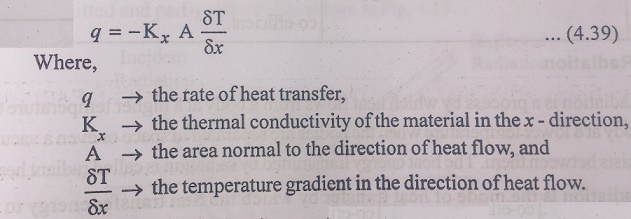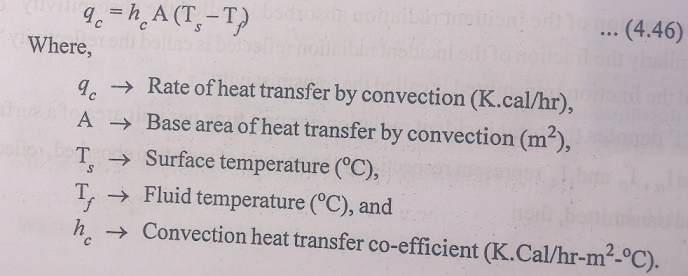Home | | Electric Energy Generation, Utilization and Conservation | Physical Principles of the Conversion of Solar Radiation Into Heat

# Physical Principles of the Conversion of Solar Radiation Into Heat

To estimate the size, the efficiency and the cost of equipment necessary to transfer a specified amount of heat in a given time, a heat transfer analysis must be made.

PHYSICAL PRINCIPLES OF THE CONVERSION OF SOLAR RADIATION INTO HEAT

To estimate the size, the efficiency and the cost of equipment necessary to transfer a specified amount of heat in a given time, a heat transfer analysis must be made. The dimensions of a solar collector, a heat exchanger or a refrigerator depend not so much on the amount of heat to be transmitted but rather on the rate at which heat is to be transferred under given external conditions. From an engineering view point, the determination of the rate of heat transfer at a specified temperature difference is the key problem in sizing a solar collector to provide a given temperature in a home or building.

Heat transfer occurs mainly by three mechanisms. The first is by conduction through solid materials in the presence of a temperature difference. The second mechanism is radiation in which energy moves in space by electromagnetic waves. In a moving fluid, the fluid molecules gain heat or lose it by conduction or radiation and carry it by their movement from one place to another. This process, the third mechanism is called convection. The heat transfer may be accompanied by other physical phenomena such as heat generation with in the medium, vapour condensation, liquid evaporation etc.

## 1. Conduction

The phenomenon of heat conduction is a process of propagation of energy between the particles of a body which are indirect contact and have different temperatures.

The Basic equation for steady state heat conduction is known as FourierŌĆÖs equation. According to this the quantity o heat(dQ), passing through an isothermal surface(dA), per time interval (dt) is proportional to the temperature gradient(╬┤T/╬┤n) and can mathematically expressed as, dQ = -K(╬┤T/╬┤n).dA dt.

Where, the proportionality factor ŌĆśKŌĆÖ is the physical property of the substance, which deines the ability of the substance to conduct heat and is called thermal conductivity. The heat flux q, defines as the rate of heat flow per unit area of the isothermal surface is given by,The direction of heat flux q is normal to the surface and is positive in the direction of decreasing temperature, which explains the negative sign on the right handside of equation.Radiation is a process by which heat flows from a body at a higher temperature to a body at a lower temperature when the bodies are separated in space or even a vacuum exists between them. The heat energy transmitted by radiation is called radiant heat.

Radiation is the mode of heat transfer by which the Sun transfer energy to the Earth. The quantity of energy leaving a surface as radiant heat depends on the absolute temperature and the nature of the surface.

A perfect radiator, so called black body emits radiant energy from its surface at a rate ŌĆśqŌĆÖ given by,Real bodies do not meet the specifications of an ideal radiator and emit radiation at a lower rather than do black bodies. The ratio of the radiation emission of a real body to the radiation emission of a black body at same temperature is called the emittance. Thus  real body emits radiation at a rate.## 3. Convection

Convection is a process that transfer heat from one region to another by motion of a fluid. The rate of heat transfer by convection qc, between a surface and a fluid can be calculated from the relation.Study Material, Lecturing Notes, Assignment, Reference, Wiki description explanation, brief detail
Electric Energy Generation and Utilisation and Conservation : Solar Radiation and Solar Energy Collectors : Physical Principles of the Conversion of Solar Radiation Into Heat |